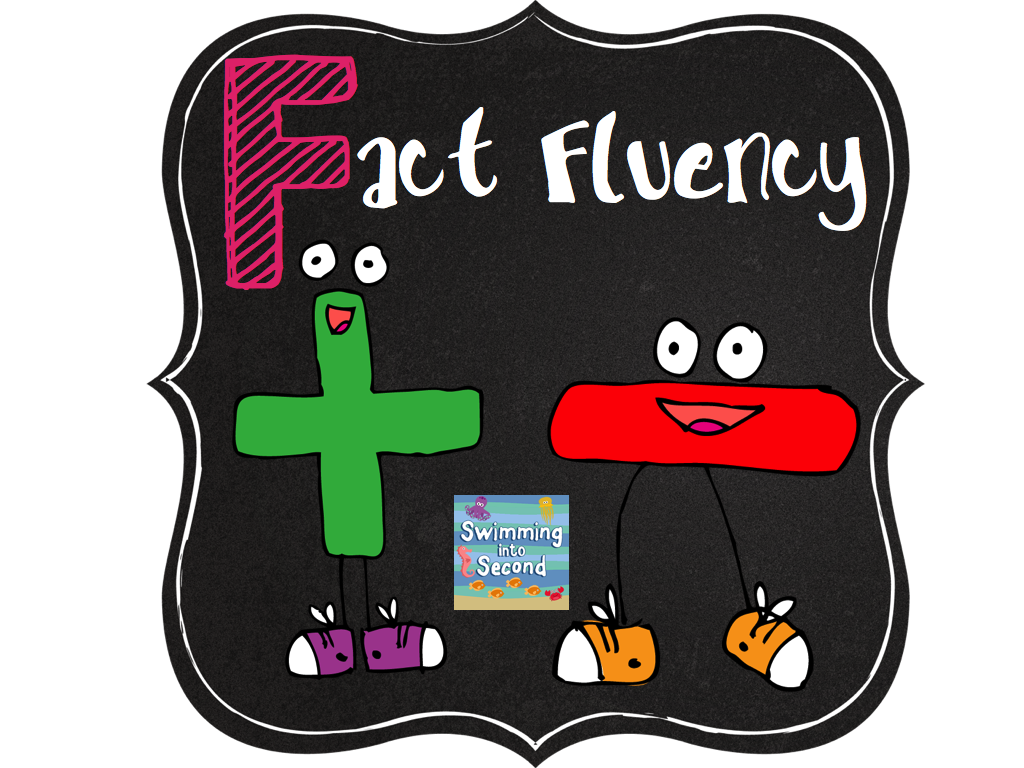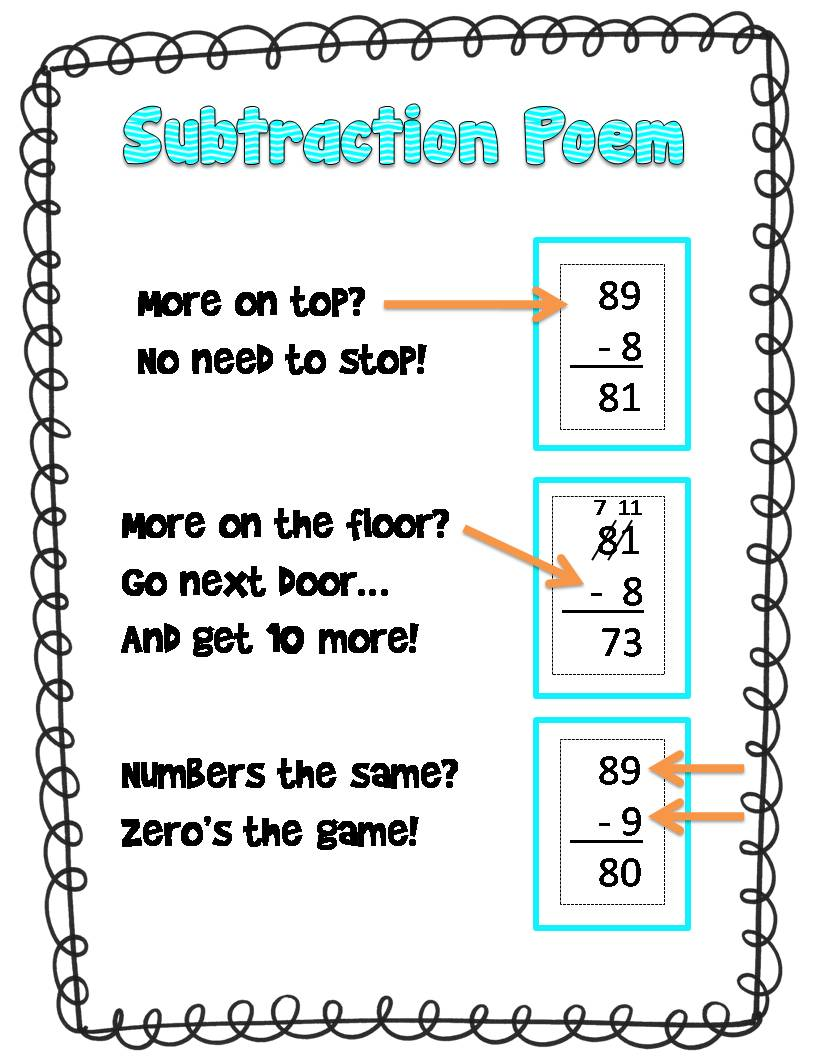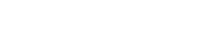Ms.  Karson  Crisp270-887-7270Email

View All Teachers

 Just For Fun!(Archived)
 FILTER BY SUBJECT:
Fun Fluency!
Due Date: 5/28/2017A fun way to practice addition strategies would be to make flash cards! These facts are so important for your student to know by heart.  Making flash cards would be a fun way to practice fact fluency!

Making Ten Strategy:

1 + 9 = 10

2 + 8 = 10

3 + 7 = 10

4 + 6 = 10

5 + 5 = 10

6 + 4 = 10

7 + 3 = 10

8 + 2 = 10

9 + 1 = 10

10 + 0 = 10

Doubles Strategy:

1 + 1 = 2

2 + 2 = 4

3 + 3 = 6

4 + 4 = 8

5 + 5 = 10

6 + 6 = 12

7 + 7 = 14

8 + 8 = 16

9 + 9 = 18

10 + 10 = 20

Doubles Plus 1 Strategy:

1 + 2 = 3

2 + 3 = 5

3 + 4 = 7

4 + 5 = 9

5 + 6 = 11

6 + 7 = 13

7 +  8 = 15

8 + 9 = 17

9 + 10 = 19

Subtraction with (and without) Regrouping!
Due Date: 5/28/2017

Your student has been learning about subtraction with and without regrouping!  I put a "how to" picture below so that you and your student can practice at home.  :)If you have any questions or concerns about the content on this page, please call Millbrooke for more information. Copyright © 2020 Millbrooke Elementary School